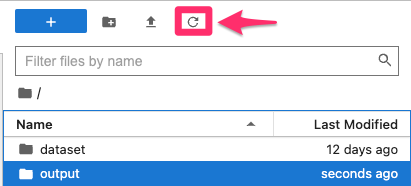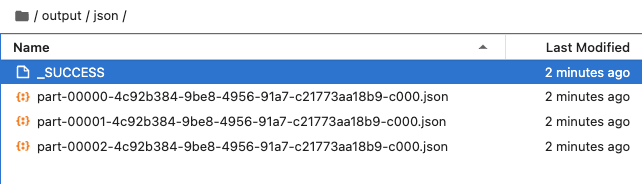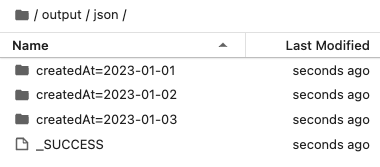# 零經驗也可的 PySpark 教學 - 資料輸出 (DataFrame writer)

Posted on  Feb 6, 2023  in  Python 模組/套件推薦 , Python 程式設計 - 高階  by  Amo Chen  ‐ 4 min read

### 啟動 PySpark Notebook Docker Container 指令 #

``````\$ docker run -it -p 8888:8888 -v \$PWD:/home/jovyan jupyter/pyspark-notebook
``````

### DataFrameWriter #

PySpark 將所有的輸出功能都整合到 pyspark.sql.DataFrameWriter ，目前該類別支援以下 5 種輸出格式：

1. Avro 是有定義結構(schema)而且以二進制儲存的格式
2. Parquet 與 ORC 都屬於 Columnar 格式，關於何謂 Columnar 格式，可以參閱這篇文章

### 輸出範例 #

``````from datetime import date
from pyspark.sql import SparkSession
from pyspark.sql.types import StructType, StructField, IntegerType, DateType

spark = SparkSession.builder.getOrCreate()

schema = StructType([
StructField('id', IntegerType(), False),
StructField('cost', IntegerType(), False),
StructField('createdAt', DateType(), False),
])

spark = SparkSession.builder.getOrCreate()
df = spark.createDataFrame([
{"id": 1, "cost": 100, "createdAt": date(2023, 1, 1)},
{"id": 2, "cost": 200, "createdAt": date(2023, 1, 2)},
{"id": 3, "cost": 250, "createdAt": date(2023, 1, 3)},
{"id": 4, "cost": 250, "createdAt": date(2023, 1, 3)},
], schema=schema)
``````

``````df.printSchema()
``````

• id
• cost
• createdAt
``````root
|-- id: integer (nullable = false)
|-- cost: integer (nullable = false)
|-- createdAt: date (nullable = false)
``````

``````type(df.write)
``````

#### JSON #

``````df.write.json('<資料夾路徑>')
``````

``````df.write.json('./output/json')
```````part-` 開頭的資料就是被輸出的 DataFrame 資料。

`_SUCCESS` 檔案代表寫入成功。實務上，如果想自動化檢查檔案是否輸出成功，可以檢查 `_SUCCESS` 檔案是否存在，如果該檔案不存在，就代表輸出失敗，即使有 `part-` 開頭的檔案存在也不應該繼續使用。

JSON 格式預設是 JSON lines, 也就是檔案中的每 1 行都是一個完整的 JSON object, 所以如果要用 Jupyter 打開輸出的檔案要選 Editor 才行。``````AnalysisException: path file:/home/jovyan/output/json already exists.
``````

``````df.write.json('./output/json', mode='overwrite')
``````

``````df.write.parquet('./output/parquet', mode='overwrite')
``````

### 為什麼 Spark 輸出多個檔案？ #

``````df.rdd.getNumPartitions()
``````

``````4
``````

#### partition() #

partition 數量可以呼叫 pyspark.sql.DataFrame.repartition 進行設定，例如將 partition 設定為 3 個：

``````df = df.repartition(3)
``````

repartition() 方法也可以按照特定欄位，切割 partition, 例如按照 `createdAt` 欄位切割為 3 份：

``````df = df.repartition(3, "createdAt")
``````

``````df = df.repartition(3, "createdAt", "cost")
``````

``````df.rdd.getNumPartitions()
# 本文結果為 3
``````

``````df.write.json('./output/json', mode='overwrite')
``````#### partitionBy() #

``````df.write.partitionBy("createdAt").json('./output/json', mode='overwrite')
``````

p.s. `partitionBy()` 方法也與 `repartition()` 相同支援多個欄位的用法``````df.withColumn('_createdAt', df.createdAt)\
.write.partitionBy('_createdAt')\
.json('./output/json', mode='overwrite')
``````

### 總結 #

pyspark.sql.DataFrameWriter — PySpark 3.3.1 documentation